Latest Banking jobs   »

# Reasoning Ability Quiz For IDBI AM/ Bank of India PO 2023 -16th March

Direction (1-5): Study the following information carefully and answer the given questions.
There are eight members in a family i.e., J, K, L, M, N, O, P and Q. There are four females and two married couples. O has one daughter and one son. P is son of K who is daughter of Q. O is father-in-law of J. M is niece of N who is brother-in-law of J. L is mother of M.

Q1. Who among the following is sister of N?
(a) L
(b) M
(c) N
(d) P
(e) None of these

Q2. Which of the following is not true about L?
(a) L is aunt of P
(b) M is daughter L
(c) L is sister of N
(d) L is grandson of O
(e) None of true

Q3. How O is related to son in law of Q?
(a) Father
(b) Daughter
(c) Son
(d) Sister-in-law
(e) Wife

Q4. How K’s sister-in-law is related to P’s father?
(a) Niece
(b) Nephew
(c) Son
(d) Daughter
(e) Sister

Q5. If Z is the wife of O. X is the son of K. How is Z related to X?
(a) Grandfather
(b) Grandmother
(c) Brother-in-law
(d) Cousin
(e) Uncle

Direction (6-10): Study the following information carefully and answer the given questions.
There are nine members in three generation family i.e., J, T, U, C, M, Q, E, S, and G. Among them there are 3 married couples and only four females. J is paternal uncle of Q. U has only 2 children. S is daughter-in-law of M and M is son-in-law of T. C is married to M. E and G are sons of C. E is not married. T is father-in-law of S’s father-in-law. T is married to U.

Q6. Who among the following person is father of Q?
(a) T
(b) U
(c) G
(d) S
(e) M

Q7. How is G related to T?
(a) Grandson
(b) Daughter
(c) Daughter in law
(d) Sister
(e) None of these

Q8. Which of the following is true about U?
(a) U is aunt of Q
(b) M is daughter of U
(c) J is sister of U
(d) S is grandson of U
(e) None of true

Q9. How S is related to son in law of T?
(a) Father
(b) Daughter in law
(c) Son
(d) Sister-in-law
(e) Wife

Q10. How M’s sister-in-law is related to J?
(a) Son in law
(b) Nephew
(c) Niece
(d) Daughter in law
(e) Sister-in-law

Directions (11-15): These questions are based on the following alphabet and digit series.
D 5 P K L 8 Z M C I 6 O Q U E 1 T N R 4 G B A 9 F S W 2 H J V 7 Y X 3

Q11. How many digits are there in above arrangement which is immediately followed by vowel?
(a) Two
(b) Four
(c) One
(d) Three
(e) None

Q12. If all the vowels are deleted from the above arrangement, then which of the following letter/digit is 10th to left of 5th from the right end?
(a) R
(b) 4
(c) G
(d) 9
(e) None of these

Q13. Which of the following letter/digit is 7th to the right of 13th from the right end?
(a) A
(b) 2
(c) H
(d) 9
(e) None of these

Q14. How many consonants are there in above arrangement which is immediately preceded by consonant and immediately followed by vowel?
(a) Four
(b) Three
(c) Two
(d) One
(e) None

Q15. Which of the following element is exactly between ‘B’ and ‘2’ in the given arrangement?
(a) 9
(b) F
(c) S
(d) W
(e) None of these

Solutions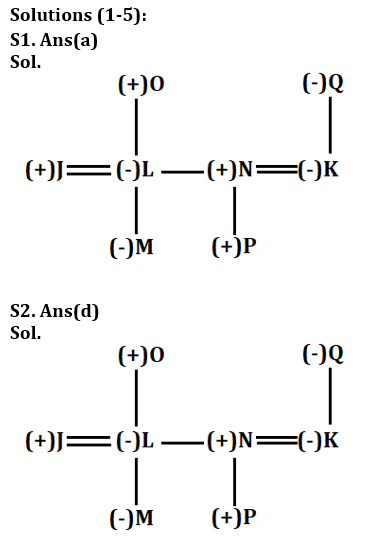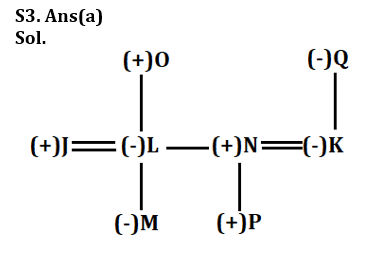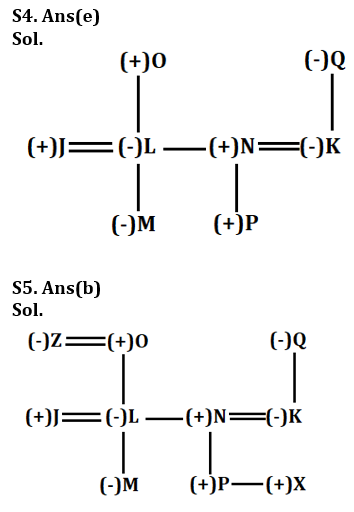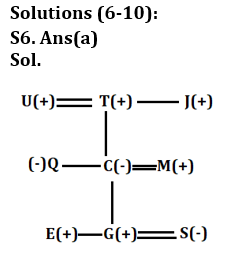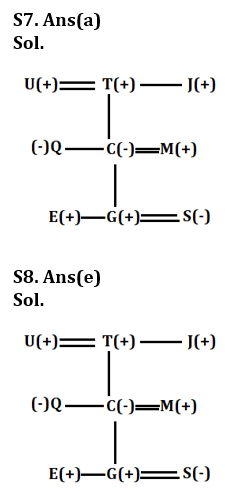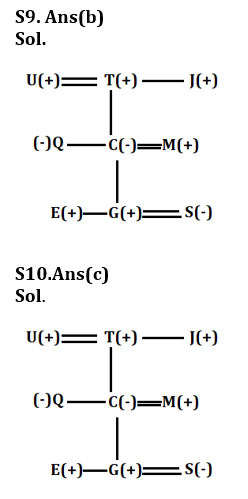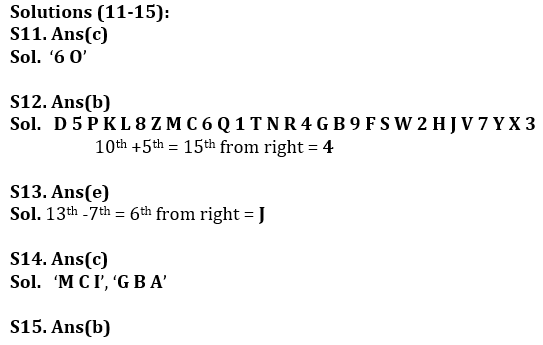## FAQs

### What is the selection process of the IDBI AM?

The selection process of IDBI AM is Online exam and Interview.

#### Congratulations!Union Budget 2023-24: Free PDF